Integer Exponents | Math for kids Grade 6, 7, & 8
1%
It was processed successfully!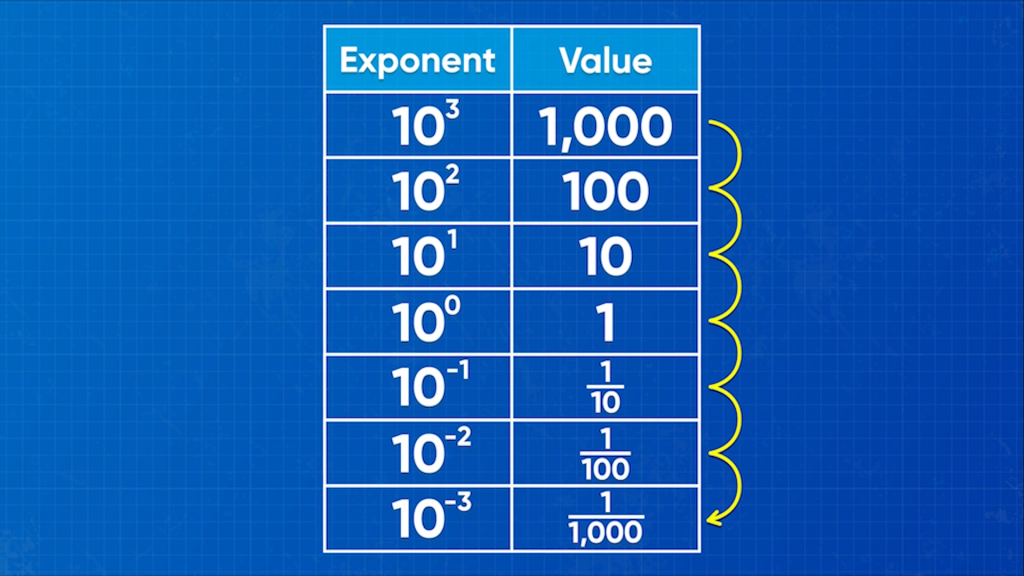WHAT IS AN EQUIVALENT EXPRESSION?

Equivalent expressions are expressions have the same value. You can use the rules of integer exponents to write expressions with exponents in simpler ways.

To better understand integer exponents…

WHAT IS AN EQUIVALENT EXPRESSION?. Equivalent expressions are expressions have the same value. You can use the rules of integer exponents to write expressions with exponents in simpler ways. To better understand integer exponents…

## LET’S BREAK IT DOWN!

### Exponents represent repeated multiplication.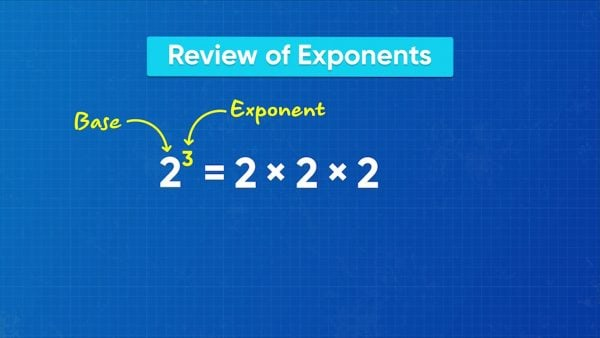An exponential expression has a base and a power. In 23, 2 is the base and 3 is the power. The base tells you the number that is multiplied. The power tells you how many times to multiply the base. So, 23 = 2 × 2 × 2. Similarly, 34 = 3 × 3 × 3 × 3. Now you try: Rewrite 25as a multiplication expression.

Exponents represent repeated multiplication. An exponential expression has a base and a power. In 23, 2 is the base and 3 is the power. The base tells you the number that is multiplied. The power tells you how many times to multiply the base. So, 23 = 2 × 2 × 2. Similarly, 34 = 3 × 3 × 3 × 3. Now you try: Rewrite 25as a multiplication expression.

### Negative exponents represent fractions.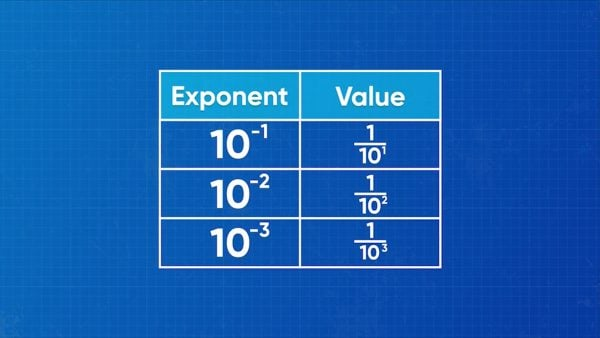Consider the following equations. 103 = 10 × 10 × 10. 102 = 10 × 10. 102 is ten times less than 103. 101 = 10. 101 is ten times less than 102. 100 = 1, which is ten times less than 101. To continue this pattern, 10–1 must be ten times less than 1, which is [ggfrac]1/10[/ggfrac]. 10–2 =[ggfrac] 1/10 × 10[/ggfrac], which is [ggfrac]1/100[/ggfrac]. 10–3 = [ggfrac]1/10× 10× 10[/ggfrac], which is [ggfrac]1/1,000[/ggfrac]. An exponent with a negative power represents 1 over the same exponent with a positive power. 10–2 = [ggfrac]1/102[/ggfrac]. This works for all negative exponents, such as 2–6 = [ggfrac]1/26[/ggfrac]. Now you try: Rewrite 3–4 as an exponent with a positive power.

Negative exponents represent fractions. Consider the following equations. 103 = 10 × 10 × 10. 102 = 10 × 10. 102 is ten times less than 103. 101 = 10. 101 is ten times less than 102. 100 = 1, which is ten times less than 101. To continue this pattern, 10–1 must be ten times less than 1, which is [ggfrac]1/10[/ggfrac]. 10–2 =[ggfrac] 1/10 × 10[/ggfrac], which is [ggfrac]1/100[/ggfrac]. 10–3 = [ggfrac]1/10× 10× 10[/ggfrac], which is [ggfrac]1/1,000[/ggfrac]. An exponent with a negative power represents 1 over the same exponent with a positive power. 10–2 = [ggfrac]1/102[/ggfrac]. This works for all negative exponents, such as 2–6 = [ggfrac]1/26[/ggfrac]. Now you try: Rewrite 3–4 as an exponent with a positive power.

### Use negative exponents to find the length of an amoeba.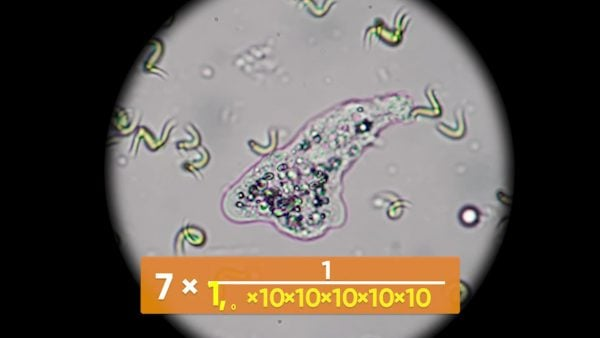An amoeba has length 7 × 10–6 m. First, evaluate the exponent: 10–6 = [ggfrac]1/106[/ggfrac] = [ggfrac]1/1,000,000[/ggfrac]. That's one millionth. 7 × one millionth is 7 millionths. The amoeba is 7 millionths of a meter long.

Use negative exponents to find the length of an amoeba. An amoeba has length 7 × 10–6 m. First, evaluate the exponent: 10–6 = [ggfrac]1/106[/ggfrac] = [ggfrac]1/1,000,000[/ggfrac]. That's one millionth. 7 × one millionth is 7 millionths. The amoeba is 7 millionths of a meter long.

### Multiply and divide exponential expressions with integer exponents.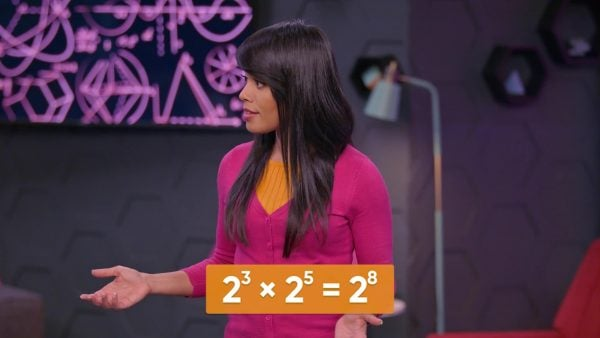Previously, you learned that when you multiply exponential expressions with the same base, you can add the powers. For example, 23 × 25 = 23+5 = 28. The same rule applies when any of the powers are negative. 4-3× 48 = 4-3+8 = 45. When you divide exponential expressions with the same base, you can subtract the powers. This rule also works with positive and negative powers. For example, [ggfrac]74/72[/ggfrac]=7-4-2=7-6.

Multiply and divide exponential expressions with integer exponents. Previously, you learned that when you multiply exponential expressions with the same base, you can add the powers. For example, 23 × 25 = 23+5 = 28. The same rule applies when any of the powers are negative. 4-3× 48 = 4-3+8 = 45. When you divide exponential expressions with the same base, you can subtract the powers. This rule also works with positive and negative powers. For example, [ggfrac]74/72[/ggfrac]=7-4-2=7-6.

### Many careers use negative exponents.Here are three examples from many careers that use negative exponents. Microbiologists use negative exponents to represent the size of very small organisms, like bacteria. Veterinarians can represent the weight of very light animals using negative exponents. Chemists use negative exponents to represent the weights of elements and compounds.

Many careers use negative exponents. Here are three examples from many careers that use negative exponents. Microbiologists use negative exponents to represent the size of very small organisms, like bacteria. Veterinarians can represent the weight of very light animals using negative exponents. Chemists use negative exponents to represent the weights of elements and compounds.

## INTEGER EXPONENTS VOCABULARY

Exponential expression
An exponential expression is another way of showing repeated multiplication. 23 = 2 × 2 × 2.
Base
In an exponential expression, the base represents the number that is being multiplied. In 23, the 2 is the base.
Power
In an exponential expression, the power represents the number of times the base is multiplied by itself. In 23, the 3 is the power.
Product of powers rule
A rule of arithmetic with exponential expressions. It says that when you multiply two exponential expressions with the same base, you can keep the base and add the exponents.
Quotient of powers rule
A rule of arithmetic with exponential expressions. It says that when you divide two exponential expressions with the same base, you can keep the base and subtract the exponents.
Equivalent expression
Two expressions are equivalent if they represent the same quantity. For example, 23and 2 × 2 × 2 are equivalent expressions because they are both equal to 8.

## INTEGER EXPONENTS DISCUSSION QUESTIONS

### What is the relationship between the following expressions: 23, 22, 21, 20, 2–1, 2-2

Each exponential expression is 12 the value of the one before it: 8, 4, 2, 1, [ggfrac]1/2[/ggfrac], [ggfrac]1/4[/ggfrac].

### What is the rule to rewrite an exponential expression with a negative power as a rational number?

A number raised to a negative power is the same as 1 over that same number raised to the positive power.

### An atom weighs 2 × 10–23 grams. How can you write that as an exponential expression with a positive exponent?

2 ×[ggfrac]1/1023[/ggfrac] or [ggfrac]2/1023[/ggfrac]

### How does the product of powers rule change when one or more powers are negative?

The rule doesn’t change. Keep the same base and add the powers. The only change is that I may be adding a negative number.

### How does the quotient of powers rule change when one or more powers are negative?

The rule doesn’t change. Keep the same base and subtract the powers. The only change is that I may be subtracting a negative number.
X

## Success

We’ve sent you an email with instructions how to reset your password.
Ok